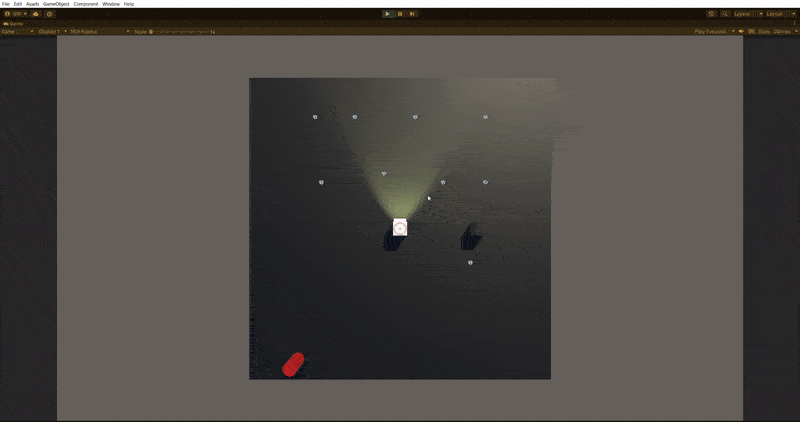# Radial UI Image is not aligned with mouse position?

I’m making a radial aim indicator on my UI canvas, and after taking pieces of various support threads and tutorials online, I finally got it to behave in the way I would like. However, its alignment with my mouse position is slightly off. I figure it has something to do with my math not lining up because I’ll admit, I’m in over my head with the math I put together to make this. I’ll show you a gif of the end result as well as the code (it’s all inside one script) and I would really appreciate if someone could point out the mistake I’ve made or the solution. The anchor in RectTransform in the Inspector is set to the centre.``````using System.Collections;
using System.Collections.Generic;
using UnityEngine;
using UnityEngine.UI;

public class UI_AimReticle : MonoBehaviour
{
public Image aimRender;
public Vector2 mouseInput;
public float positionOffsetx = 150f;
public float positionOffsety = 150f;
public Vector2 _centre;

void Awake()
{
// float aimHorizontal = Input.GetAxis("Third Axis");
// float aimVertical = Input.GetAxis("Fourth Axis");
_centre = Vector2.zero;

}

void FixedUpdate()
{
if (Input.GetMouseButton(1))
{
aimRender.color = new Color(1, 1, 1, 0.67f);
mouseInput.x = Input.mousePosition.x - (Screen.width / 2f);
mouseInput.y = Input.mousePosition.y - (Screen.height / 2f);
mouseInput.Normalize();
float angle = Mathf.Atan2(-mouseInput.y, mouseInput.x);
// angle *= 180;
angle += 90f;
if (angle < 0)
{
angle += 360;
}
var x = Mathf.Sin(angle) * positionOffsetx;
var y = Mathf.Cos(angle) * positionOffsety;
RectTransform reticle = aimRender.GetComponent<RectTransform>();
reticle.anchoredPosition = _centre + new Vector2(x, y);
}
else
{
aimRender.color = new Color(1, 1, 1, 0);
}

}

}

``````